# Force and Motion Force and Motion A force

• Slides: 21Force and MotionForce and Motion • A force is a push or pull exerted on an object. • A force exerted on an object causes that object’s velocity to change, which causes an acceleration. • The symbol F is a vector and represents the size and direction of a force.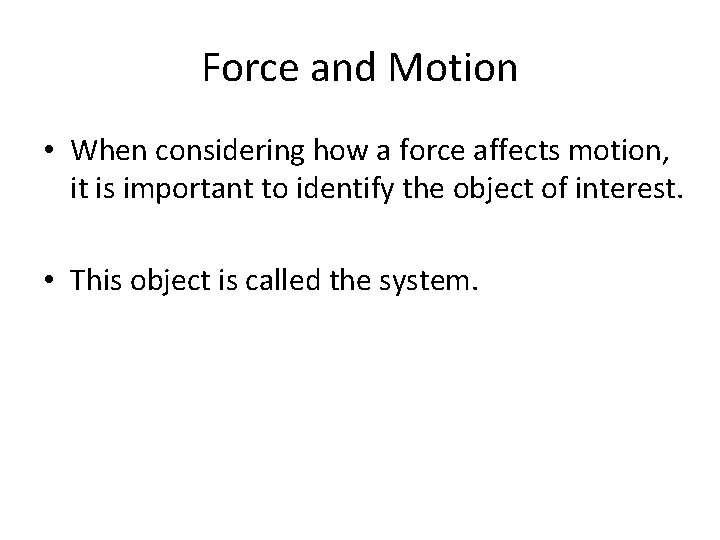Force and Motion • When considering how a force affects motion, it is important to identify the object of interest. • This object is called the system.Contact Forces and Field Forces • Forces result from interactions which makes each force has a specific and identifiable cause called the agent. – Ex: when you push your textbook, your hand (the agent) exerts a force on the textbook (the system).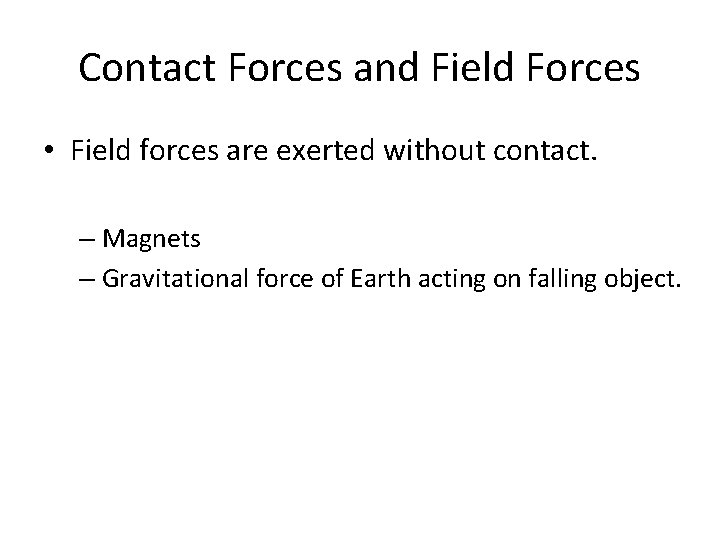Contact Forces and Field Forces • Field forces are exerted without contact. – Magnets – Gravitational force of Earth acting on falling object.Free-Body Diagrams • Sketch the situations and circle the system. • Identify every place where the system touches the external world. It is at these places the contact forces are exerted. • Identify the contact forces. • Represent each force with an arrow that points in the direction that the force is applied.Free-Body Diagrams • Try to make the length of each arrow proportional to the size of the force. • Label each force.Practice • Specify the system and draw a free body diagram. Draw all forces and indicate the direction of the acceleration and of the net force. Draw vectors of appropriate lengths. – A book held in your hand – A book pushed across the desk by your hand – A book on a desk when your hand is pushing down on itForce and Acceleration • Force = acceleration x mass • F = ma • F is measured in Newtons (N) • A = F/m – Newton’s 2 nd LawCombining Forces • When forces are in opposite direction, the resulting vector is the length of the difference between the two. • When they are in the same direction, you add. • Another term for the vector sum of all the forces on an object is the net force.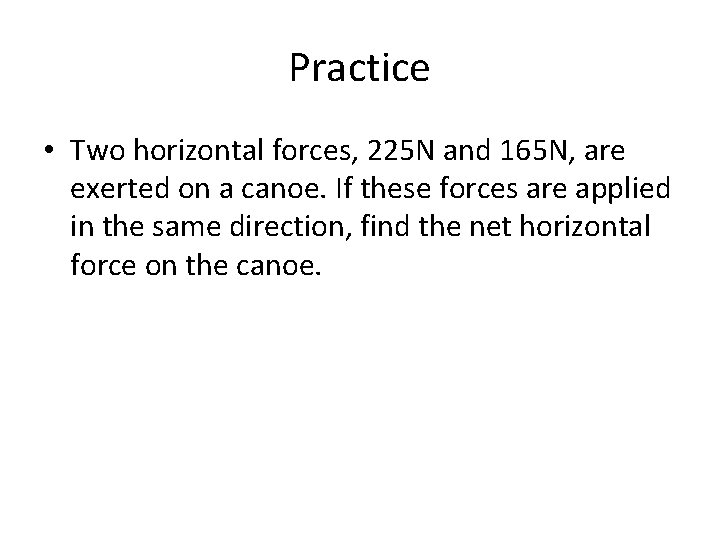Practice • Two horizontal forces, 225 N and 165 N, are exerted on a canoe. If these forces are applied in the same direction, find the net horizontal force on the canoe.Practice • If the same two forces are exerted on the canoe is opposite direction, what is the net horizontal force on the canoe? Be sure to indicate the direction of the net force.Practice • Thee sleigh dogs are pulling a sled across the Alaskan snow. Alutia pulls east with a force of 35 N, Seward also pulls east with a force of 42 N, and Kodiak pulls west with a force of 53 N. What is the net force on the sled?Practice • Calculate the force you exert as you stand on the floor ( 1 lb = 0. 454 kg). Is the force the same if you lie on the floor?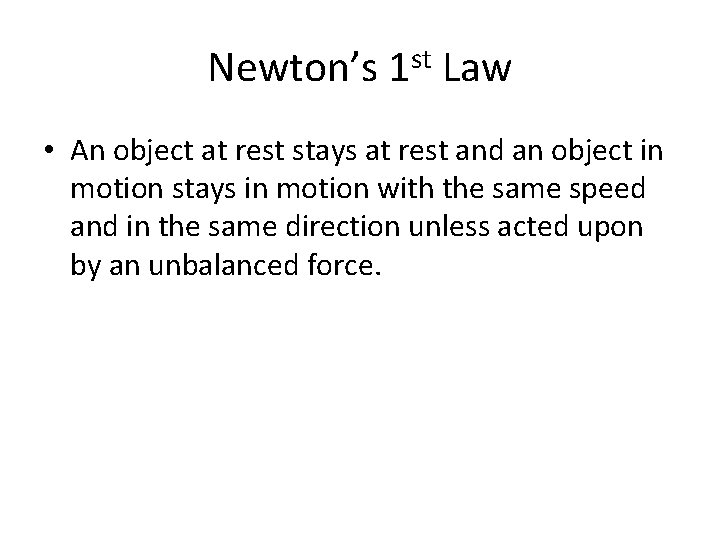Newton’s 1 st Law • An object at rest stays at rest and an object in motion stays in motion with the same speed and in the same direction unless acted upon by an unbalanced force.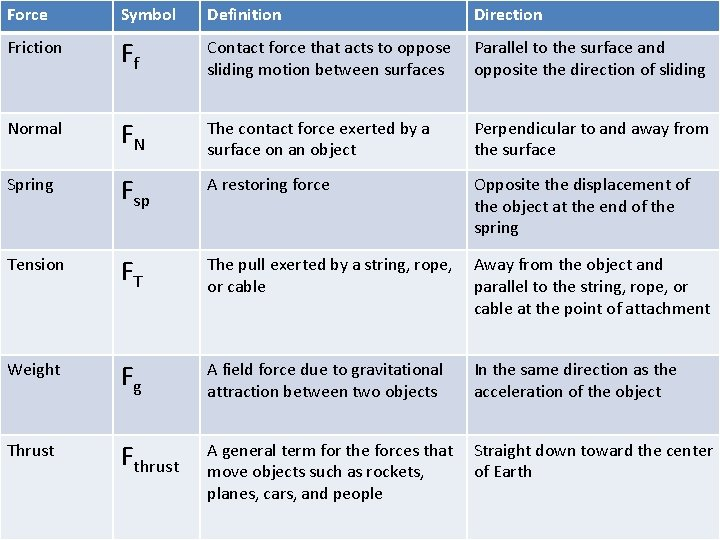Force Symbol Definition Direction Friction Ff Contact force that acts to oppose sliding motion between surfaces Parallel to the surface and opposite the direction of sliding Normal FN The contact force exerted by a surface on an object Perpendicular to and away from the surface Spring Fsp A restoring force Opposite the displacement of the object at the end of the spring Tension FT The pull exerted by a string, rope, or cable Away from the object and parallel to the string, rope, or cable at the point of attachment Weight Fg A field force due to gravitational attraction between two objects In the same direction as the acceleration of the object Thrust Fthrust A general term for the forces that move objects such as rockets, planes, cars, and people Straight down toward the center of Earth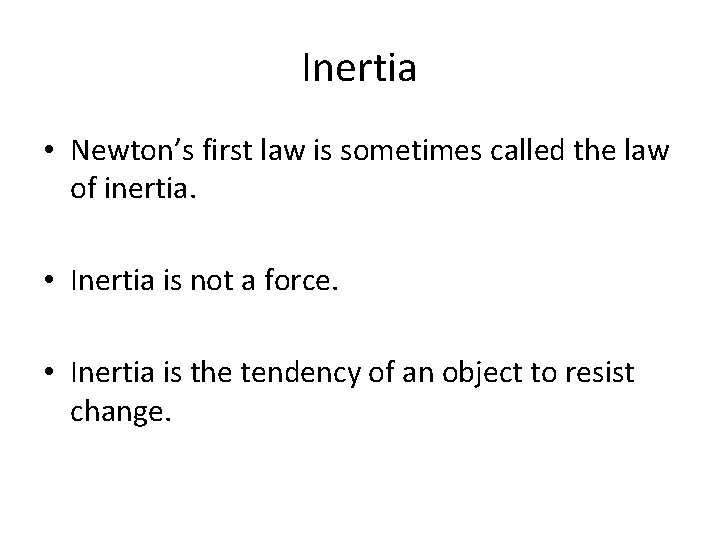Inertia • Newton’s first law is sometimes called the law of inertia. • Inertia is not a force. • Inertia is the tendency of an object to resist change.Equilibrium • If the net force on an object is zero, then the object is in equilibrium. • An object is in equilibrium if it is at rest or if it is moving at a constant velocity.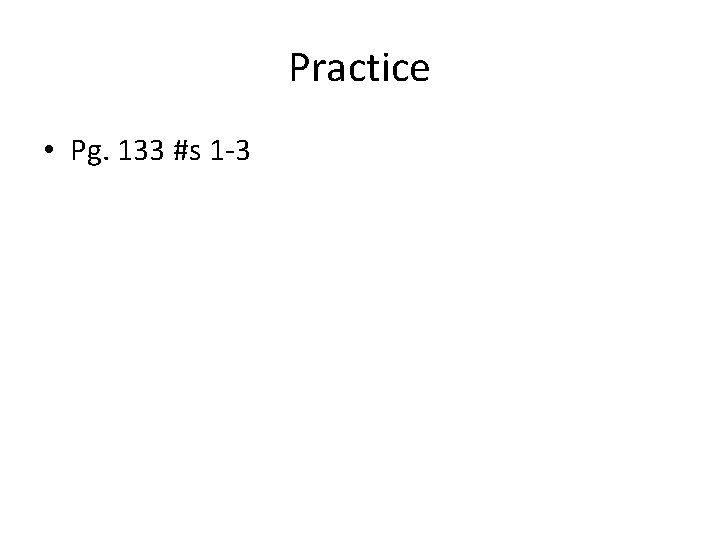Practice • Pg. 133 #s 1 -3Practice • Identify each of the following as either, a) contact force, b) a field force, c) not a force: – Weight – Mass – Inertia – Push of a hand – Thrust – Resistance – Air resistance – Spring force – acceleration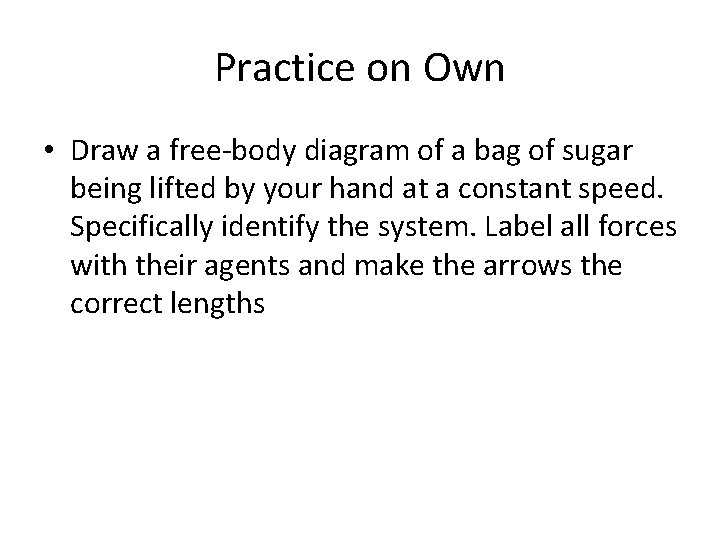Practice on Own • Draw a free-body diagram of a bag of sugar being lifted by your hand at a constant speed. Specifically identify the system. Label all forces with their agents and make the arrows the correct lengths